Next: Power and Internal Resistance Up: Electric Current Previous: Capacitors in DC Circuits

## Energy in DC Circuits

Consider a simple circuit in which a battery of voltagedrives a currentthrough a resistor of resistance. As we have seen, the battery is continuously doing work by raising the potentials of charges which flow into its negative terminal and then flow out of its positive terminal. How much work does the battery do per unit time? In other words, what is the power output of the battery?

Consider a (positive) chargewhich flows through the battery from the negative terminal to the positive terminal. The battery raises the potential of the charge by, so the work the battery does on the charge is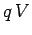. The total amount of charge which flows through the battery per unit time is, by definition, equal to the currentflowing through the battery. Thus, the amount of work the battery does per unit time is simply the product of the work done per unit charge,, and the charge passing through the battery per unit time,. In other words,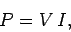(141)

where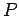, of course, stands for the power output of the battery. Thus, the rule is
The power in a DC circuit is the product of the voltage and the current.
This rule does not just apply to batteries. If a currentflows through some component of a DC circuit which has a potential dropin the direction of current flow then that component gains the energy per unit time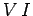at the expense of the rest of the circuit, and vice versa. Incidentally, since the SI unit of power is the watt (W), it follows that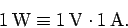(142)

Consider a resistorwhich carries a current. According to Ohm's law, the potential drop across the resistor is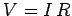. Thus, the energy gained by the resistor per unit time is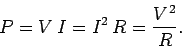(143)

In what form does the resistor acquire this energy? In turns out that the energy is dissipated as heat inside the resistor. This effect is known as Joule heating. Thus, the above formula gives the electrical heating power of a resistor. Electrical energy is converted into heat (i.e., random motion of the atoms which make up the resistor) as the electrically accelerated free electrons inside the resistor collide with the atoms and, thereby, transfer all of their kinetic energy to the atoms. It is this energy which appears as heat on a macroscopic scale (see Sect. 7.3).

Household electricity bills depend on the amount of electrical energy the household in question uses during a given accounting period, since the energy usage determines how much coal or gas was burnt on the household's behalf in the local power station during this period. The conventional unit of electrical energy usage employed by utility companies is the kilowatthour. If electrical energy is consumed for 1 hour at the rate of 1 kW (the typical rate of consumption of a single-bar electric fire) then the total energy usage is one kilowatthour (kWh). It follows that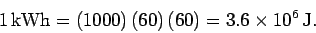(144)Next: Power and Internal Resistance Up: Electric Current Previous: Capacitors in DC Circuits
Richard Fitzpatrick 2007-07-14